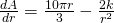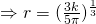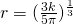# 2012 A-level H2 Mathematics (9740) Paper 1 Question 10 Suggested SolutionsAll solutions here are SUGGESTED. Mr. Teng will hold no liability for any errors. Comments are entirely personal opinions.

(i)
Volume,Surface Area,Setwhen.

Thus A is minimum when(ii)
Surface Area,Using the Graphing Calculator, we find thatSinceNotice that the value of r and h is the same. Which actually makes some intuitive sense if you attempt to visualise. Students should simplify to the simplest form, using appropriate indices formula.
For (ii), it can be solved using GC entirely. If students can, they should draw the curve out.

•pnut

Hey would just like to highlight that there is a typo for the final polynomial equation which should be 5(pi)r^3 -540r+1200=0 and at the end we need to reject r=-6.759

Thanks for the answer keys though 😀

•KS Teng

thank you 🙂

Not readable? Change text.